# TensorFlow 3. A Small and Interest word2vec NN example to start your adventure

The note intention is to understand the word2vec, and how to build a small NN to start your adventure on Deep Learning. You can see many source codes here to build the NN. But I am not yet built it with TF.

1. Reference:
1. A visual toy NN network for word2vec generation.

The whole source code is from https://ronxin.github.io/wevi/.  The source code is written by javascript for NN with html and svg (https://www.tutorialspoint.com/d3js/d3js_introduction_to_svg.htm) for graph visibility.  Try your best to understand all thoroughly (not just well enough). It will help you to master the basic spirit of NN.

1. The article mainly refers to

http://mccormickml.com/2017/01/11/word2vec-tutorial-part-2-negative-sampling/

https://cs224d.stanford.edu/lecture_notes/notes1.pdf

https://cs224d.stanford.edu/project.html

http://arxiv.org/pdf/1301.3781.pdf

https://github.com/svn2github/word2vec

https://github.com/fginter/w2v_demo

http://bionlp-www.utu.fi/wv_demo/

http://mccormickml.com/2016/04/19/word2vec-tutorial-the-skip-gram-model/

1. Some generated word2vec data

Chinese word2vec data: (open with ultraedit)

F:AllHere2014Apr12_2015Nov02personalWordEmbeddingmakeWVChinesewordvectors-masterdatazh.tsv

https://github.com/candlewill/Chinsese_word_vectors

https://github.com/fginter/wvlib_light/tree/3471a8db66883769c4e5398806876d5be3e3df24

data: http://bionlp-www.utu.fi/fin-vector-space-models/

1. What is word2vec?

“These methods were prediction based in the sense that they provided probabilities to the words and proved to be state of the art for tasks like word analogies and word similarities. They were also able to achieve tasks like King -man +woman = Queen,”

Given a word, tell the probability of another word will be appear.

For example,

*1) if I give you a word “salad”, then the probability of another word “Lettuce” is much bigger than word “shoes”.

*2) given you a word “Soviet”, the next word with much higher probability will be “Union” or “Russia” than “google”.

Also, one example in Google word2vec is able to get “King”-“man”=”Queen”. That is wonderful.

1.     Why word2vec?

Computer is good at computing with number. All things in the world need to map into a number if we want to handle it.  We have lots of coding standard for word, such as ANSI, Unicode, GB2810, etc.  For example, below are the ANSI encoding for three words:

• salad: 73 61 6C 61 64
• Lettuce 4C 65 74 74 75 63 65
• shoes: 20 73 68  6F 65 73

Of course, those coding are doing well in word processing software (such as Microsoft Word, or Google Word Doc) for you to write your essay to turn in to your teacher. However, if we want to do much more intelligent things, such as predict the next word in the voice recognition, computer with this ANSI code will not understand how to group  “73 61 6C 61 64” with “4C 65 74 74 75 63 65” closer than “20 73 68  6F 65 73”.

In AI, we have another one-hoc coding for words. We will use one binary in every position to express one word.  For example,

• Lettuce:010
• Shoes: 100

So, if you have thousands of words to process in your AI program, you will have thousands of 0 and only one 1 to represent a word.  Also, the coding is still not yet able to identify the close relationship between words.

Of course, instead of using AI NN, we can using the pure probability model to compute all the relationship between words. However, you need to use a very very huge matrix to store those relationships. It will be terrible huge, and may compute hard in the training and also in the real-time application.

Another models are Co-Occurrence Matrix as below, to count the words co-occurrence in a sentence with a window of x. It still requires huge of space to store the relationship. Of course, you can use PCA to simplify the space.

Below is the example on how to calculate the Co-Occurrence Matrix.

Corpus = He is not lazy. He is intelligent. He is smart.   (window of 2)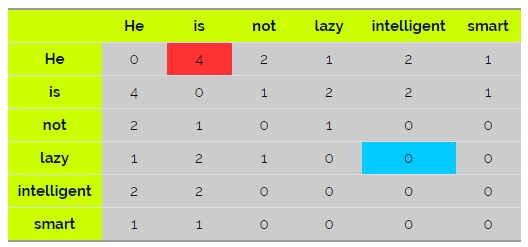1. Two models using word2vec:  The way how you create training pairs.

It is a supervised NN training. We need to provide the training data with correct pair of input and output.  For example, (salad, lettuce), (lettuce, salad) are two training pair data.

1. CBOW (Continuous Bag of Words): to predict the word with context.

For example, you are asking to fill the last word in below sentence:

I was born in China. I can speak “____”.

Yes, you will guest “Chinese” and “lettuce” in the blank, instead of “French” and “Shoes”.

1. skip-gram neural network model : to predict the context with word.

For example, in the sentence of “the cat ate the mouse”, we provide word “ate”, you need to predict the context.

Actually, if only consider on the result of training data, the two models are not so different. The final results of training data for the two models are just data pairs.

Suppose, we have a corpus C = “Hey, this is sample corpus using only one context word.” and we have defined a context window of 1.

We get below two training data sets according to the two models: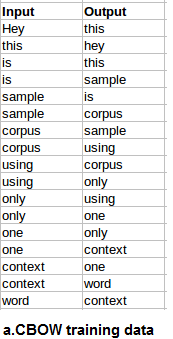Below is the explanation.

1. CBOW: target/output is center word

Here is on how to get the training pair for sentence: “Hey, this is sample corpus using only one context word.”  (windows of 1.  Left is phrase, right is the training pair)

• phrase “___ Hey this”:   (this, Hey) , ___ is a blank word, so “Hey” is center/output
• phrase “Hey, this is”:   (Hey,this), (is, this), as “this” is center/output
• phrase “this is sample”: (this, is), (sample,is) , as “is” is center/output
• phrase “context word ___”: (context,word) as “word” is center/output

1. skip-gram: target/output is context/surrounding word.
• phrase “___ Hey this”:   (Hey,this)
• phrase “Hey, this is”:   (this ,Hey), (this, is)
• phrase “this is sample”: (is ,this), (is ,sample)
•  phrase “context word ___”: (word,context)

Another good sample for skip-gram training pair as below.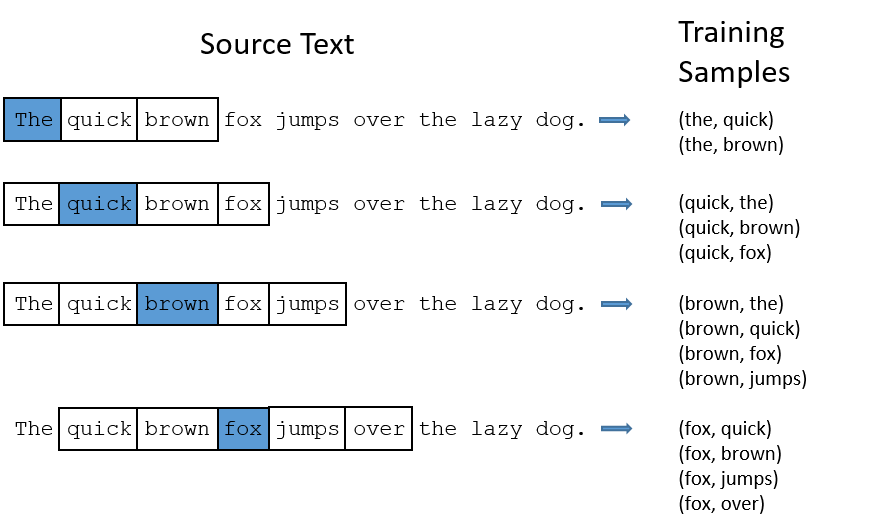After you got the training data pair (input, output), you are ready for NN now.

1.  Simple NN to generate word vector to describe the relationship between words.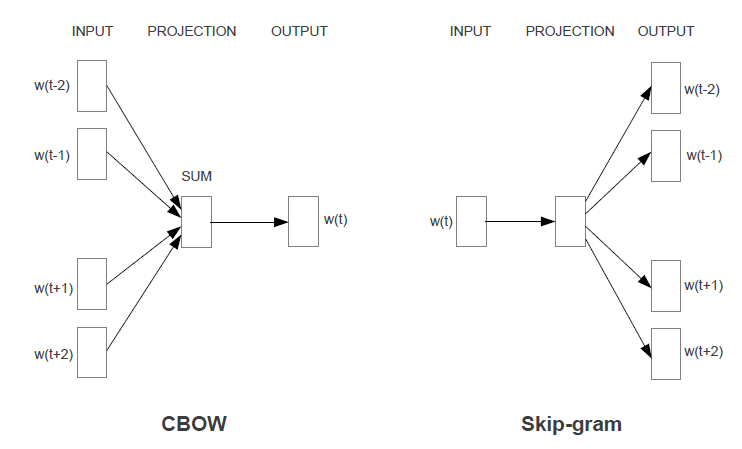1. From initial training word pair to initial vector pair.

As we known before, computer only cares number. So, word training pair needs to be number. We use one-hoc coding to transfer the training pair from word to vector number.

It is easy to turn word to vector. You can use dictionary as the reference. Assumed you have the dictionary with 50000 words. The first word, such as “a” will be “1000000…00” (total number of 0 is 49999). The 2nd word, such as “Abacus”, will be “0100000…00”. The last word, such as “zebra” will be “0000…001” (Again,the total number of 0 is 49999).

Yes. That is easy, right?

1. Build a three layers of NN: one input, one hidden and one output layer.

Below is a toy example of NN with hidden layer of 5 Neurons, 8 inputs and 8 outputs. It is to calculate the relationship among those input words.  In practice, you may build hidden layer of 100 Neurons to 1000 Neurons, with 50000 inputs and 50000 outputs.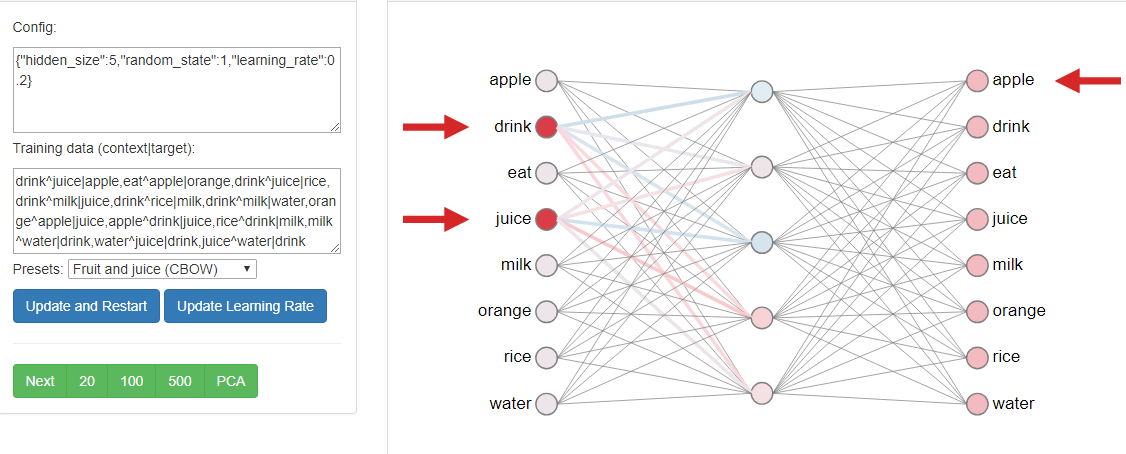Of course, the above diagram is just for human visibility.  The backend compute logic is as below.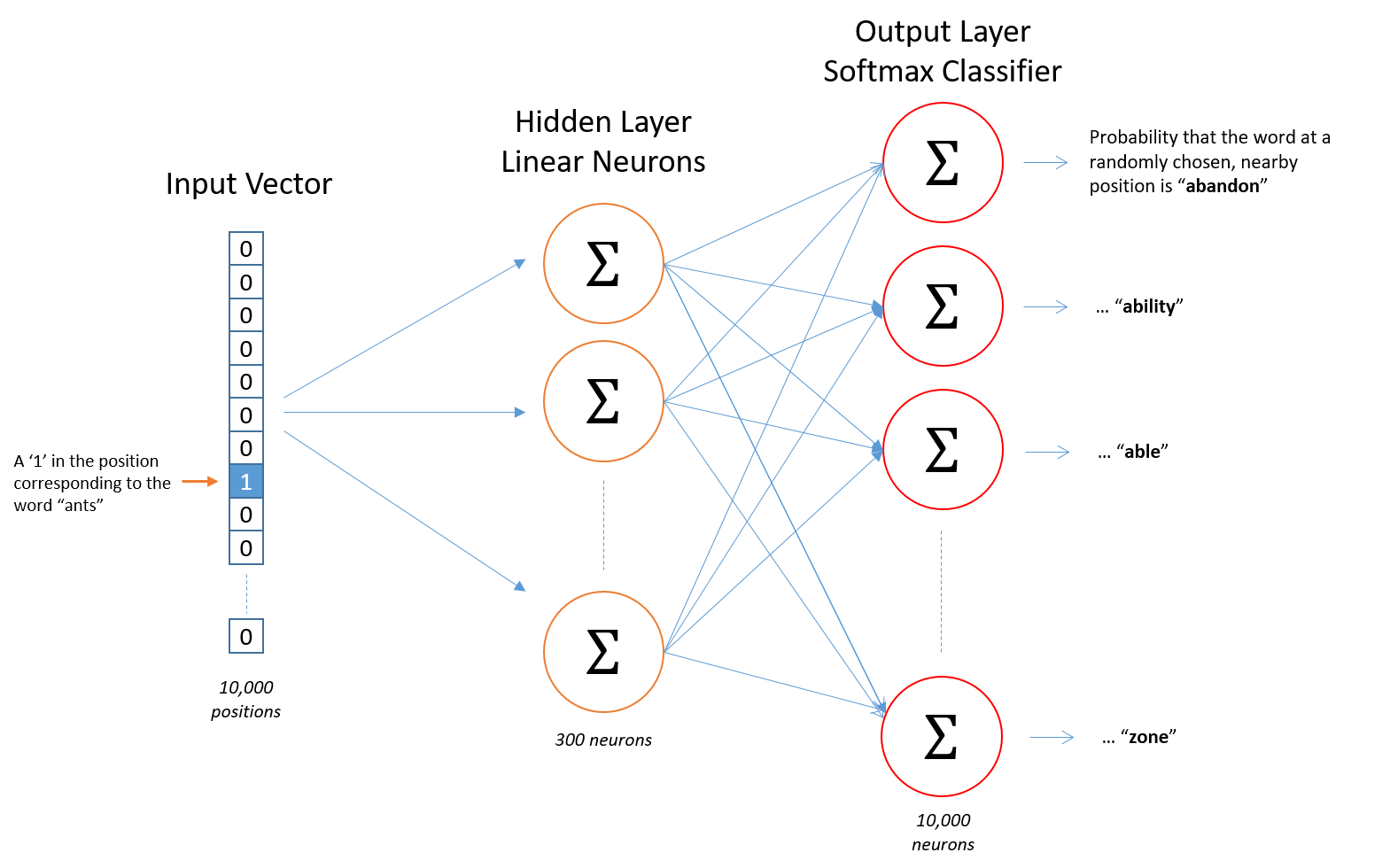1. Ok, what is the NN task?

The task is to learn the weight. So that you can generate the output layer of word vector (which is not only 0 or 1 as initial word pair. It will be the probability of each word.)1. Why we can get the optimized weight form NN?

You may refer to another note “1. Explain4GradientDescentOptimizerMinimizeByLinearRegressionExample “ for detail math logic behind the NN.

Here is the short summary.

1. First you forward to feed the input data to the NN, and calculate with bunch of weight parameters, and finally get the softmax output for the possibility of the relation of all dictionary words.

For example, we are now feeding the training pair of (apple,ipad) to computer. The actual vector pair that handle by computer maybe (00010,010000).

So, after the forward calculation, you may get a NN output vector (0.1,0.1,0.1,0.1,0.3,0.3)

1. Compare training output with NN output to update weight

We found that, the NN output=(0.1,0.1,0.1,0.1,0.3,0.3) is not same as our training output, which is 010000, that should be=(0,1,0,0,0,0) in computer.

So, we need to use the good dog and bad dog game to punish the NN, so that NN can update the weight parameters.

To do that, we need to define the cost function. We use the cost function to see how is the NN output far away from our training data output.  From other article,

“Very often when we are trying to learn a probability from some true probability, we look to information theory to give us a measure of the distance between two distributions. Here, we use a popular choice of distance/loss measure, cross entropy.”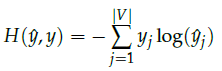Our optimized goal is to minimize the H.

As ouris full of 0, except one 1. So the ugly complex formula can be simplified to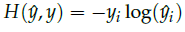. We only care the non zero index of the two vectors.

Ok, although we believe textbook are correct, however, we still need to verify by our intuitive. Let’s make an example to see why the cost function works.

Assume your NN learning output is same as the training output 010000 (the index 2 is 1). So, the H=0. Wow, that means the distance of predict and the output is 0, which will be no punish.

However, if you learning output for index 2 is 0.01, then you will get H=-1log(0,01)=4.6.  That will be a big distance between two outputs, which will generate the punish/updated request for weight parameter to make the distance shorter.

If you get huge of training data, you will finally get the much optimized weight parameter.

1. Final step: use the optimized weight to get the word vector for each word.

Just feed each word again into NN, you will get the NN output vector. The vector will be this word’s actual word vector.

1. Improvement with Negative Sampling

From above cross entropy formulate, we only use the good word pairs, which are called as positive pairs that we generate from good corpus/documents. For example,   “Hey, this is sample corpus using only one context word.” Is a good corpus. However, if you randomly pickup words from dictionary, you may form a bad corpus, such as “stock boil fish is toy”.

From bad corpus, you will generate a negative sample of word pairs. So, you may use those negative word pairs to update weight parameters if the NN output is same/similar as training output.

So, what is the cost function, “we build a new objective function that tries to maximize the probability of a word and context being in the corpus data if it indeed

is, and maximize the probability of a word and context not being in the corpus data if it indeed is not.”

1. Why use simple NN?

Can we use deep NN to provide better word2vec? That is a good question. You may try it. This maybe my next note.

« (Previous News)